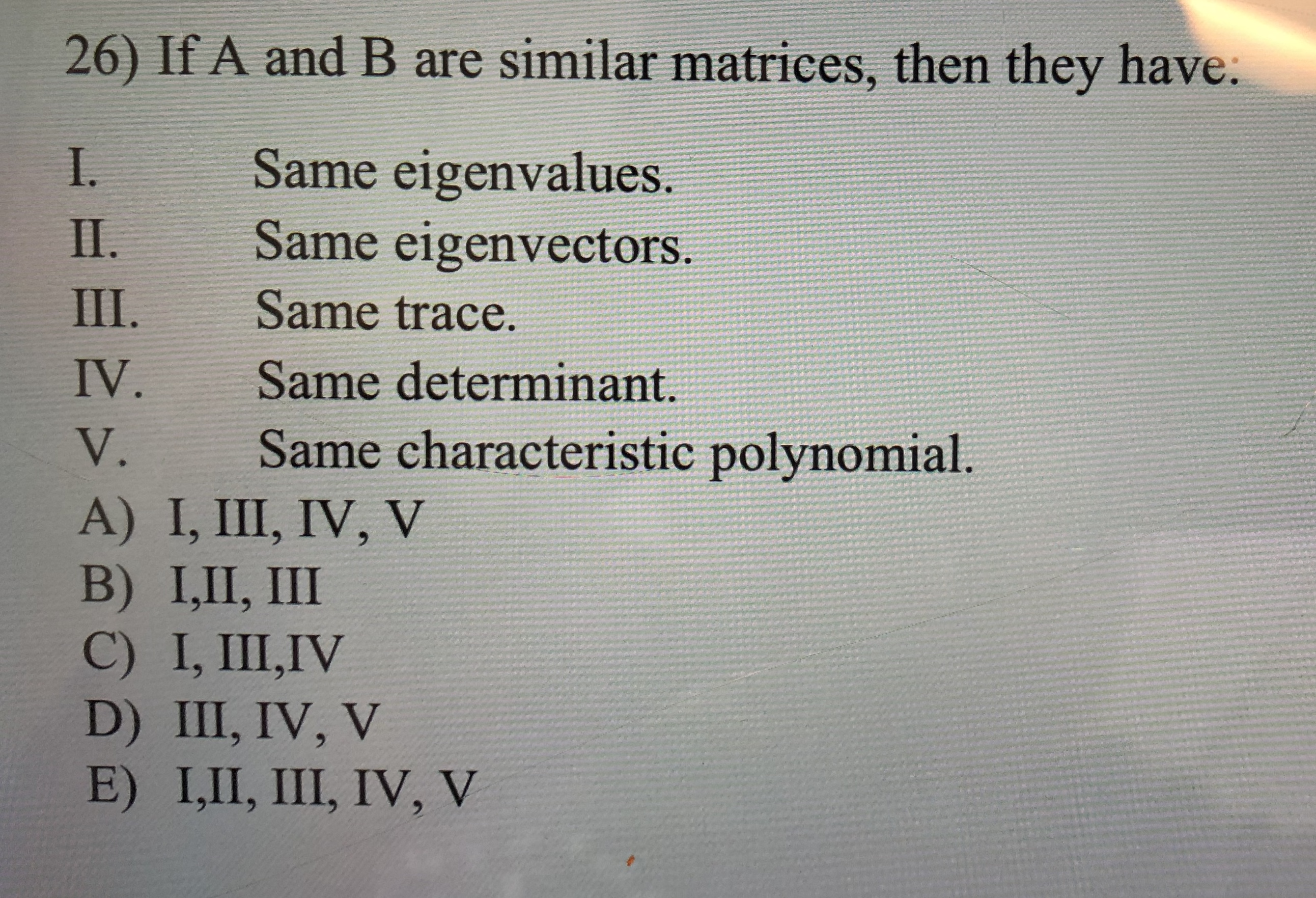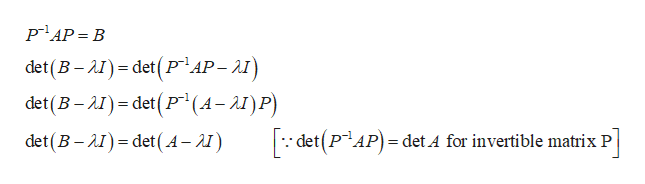# 26) If A and B are similar matrices, then they have:Same eigenvalues.Same eigenvectors.I.II.III.Same trace.IV.Same determinant.Same characteristic polynomialV.A) I, III, IV, VB) I,П, IIC) I, III,IVD) III, IV, VE) I,II, III, IV, V

Question
12 viewshelp_outlineImage Transcriptionclose26) If A and B are similar matrices, then they have: Same eigenvalues. Same eigenvectors. I. II. III. Same trace. IV. Same determinant. Same characteristic polynomial V. A) I, III, IV, V B) I,П, II C) I, III,IV D) III, IV, V E) I,II, III, IV, V fullscreen
check_circle

Step 1

Similar matrices have same determinant.

Step 2

Similar matrices then they have same trace.

Step 3

Given that A and B are similar matrices then th...help_outlineImage TranscriptionclosePAP B det (B-) det(PAP-AI) det(B-I) detP(A-)P) det (B-I det(A-) detPAP = det A for invertible matrix P = fullscreen

### Want to see the full answer?

See Solution

#### Want to see this answer and more?

Solutions are written by subject experts who are available 24/7. Questions are typically answered within 1 hour.*

See Solution
*Response times may vary by subject and question.
Tagged in

### Math